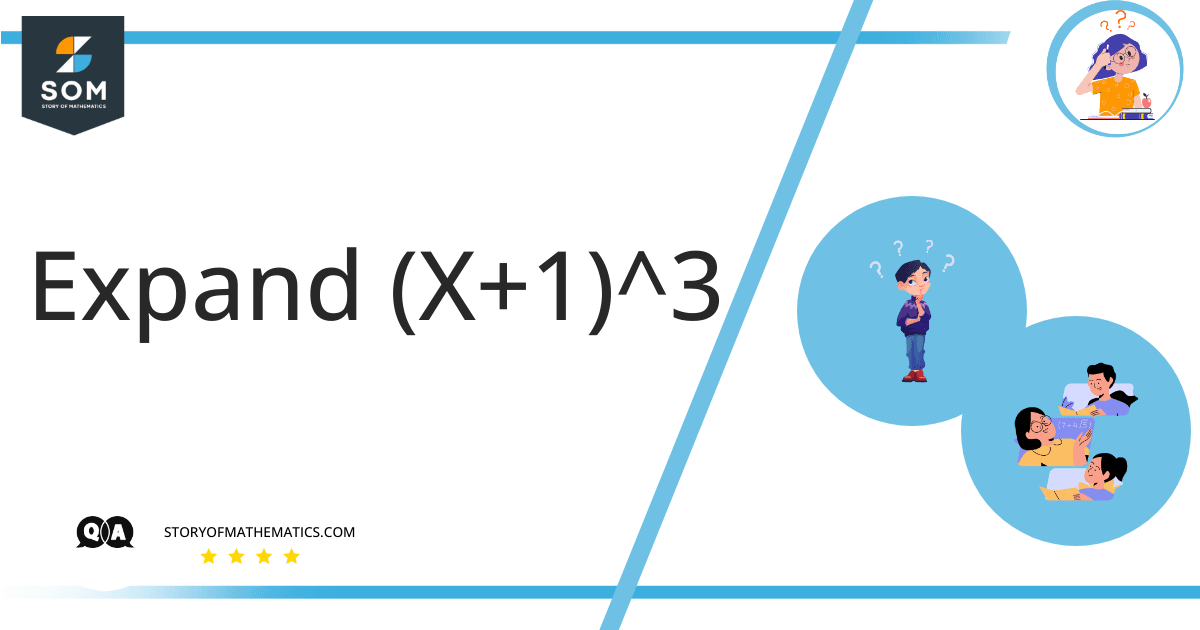# Expand the expression (x+1)^3.This question aims to find a way to expand the given expression by using a particular method.

The given expression is $( x + 1 ) ^ 3$ which is in the form of power. There is no other excellent method to calculate such expressions than using the binomial theorem. According to the binomial theorem, the expressions written in the form of $( a + b ) ^ n$, where a + b is the expression and n is the power can be expanded easily.

If the value of n is greater, the expansion of the expression becomes lengthy but it is a useful tool to calculate the expansion of expression written with large powers.

The binomial theorem is used to calculate the expressions or numbers having finite powers. The binomial theorem is not valid for infinite powers.

The binomial theorem is represented in the following way when the given expression is not in the fraction form:

$( a + b ) ^ n = a ^ n + n b ^ { n – 1 } b + \frac { n ( n – 1 ) } { 2 ! } a ^ { n – 2 } b ^ 2 + \frac { n ( n – 1 ) ( n – 2 ) } { 3 ! } a ^ { n – 3 } b ^ 3 + …. + b ^ n$

In the given expression, the value of a is x and b is -1. By putting the values in the above formula:

$( x + 1 ) ^ 3 = x ^ 3 + 3 ( x ) ^ { 2 } + \frac { 3 ( 3 – 1 ) } { 2 ! } x ^ { 3 – 2 } 1 ^ 2 + \frac { 3 ( 3 – 1 ) ( 3 – 2 ) } { 3 ! } x ^ { 3 – 3 } 1 ^ 3 + … + x ^ n$

By solving the above equation, we get:

$= x ^ 3 + 3 ( x ) ^ { 2 } + \frac { 3 ( 2 ) } { 2 ! } x ^ { 1 } + \frac { 3 ( 2 ) ( 1 ) } { 3 ! } x + …. + x ^ n$

$( x + 1 ) ^ 3 = x ^ 3 + 3 x ^ 2 + 3 x + 1$

## Numerical Results

The expansion of $( x + 1 ) ^ 3$ is $x ^ 3 + 3 x ^ 2 + 3 x + 1$.

## Example

Find the expansion of $( x + 1 ) ^ 2$.

$= x ^ 2 + 2 ( x ) ^ { 1 } x + \frac { 2 ( 1 ) } { 2 ! } -1 ^ { 2 – 2 } x ^ 2 + … + x ^ n$

$( x + 1 ) ^ 2 = x ^ 2 + 2 x ^ 2 + 1$

The expansion of the expression having power 2 is calculated as $x ^ 2 + 2 x ^ 2 + 1$ .

Image/Mathematical drawings are created in Geogebra.